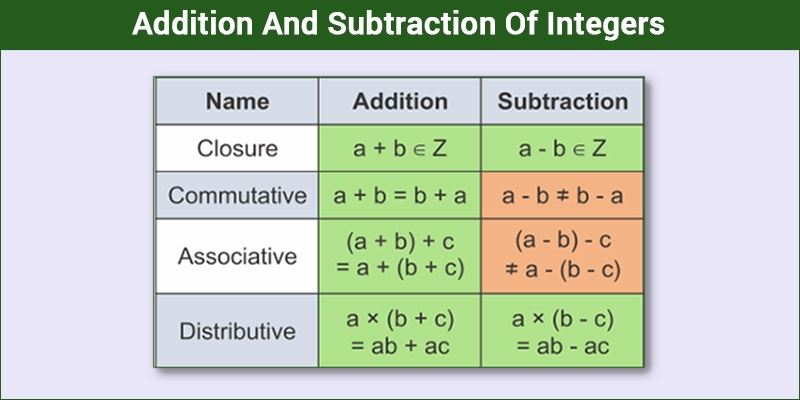# Addition And Subtraction Of Integers

Addition And Subtraction Of Integers explained here. Mathematics is a subject that deals with numbers. An arithmetic operation is an elementary branch of mathematics. Arithmetical operations include addition, subtraction, multiplication and division. It helps us to find the sum (total) or difference (how more or less) of something. Not only sum or difference, it also helps one to compare and divide things equally. Arithmetic operations are applicable to all real numbers including integers.## Addition And Subtraction Integers Rules

Integers are a special group of numbers that are positive, negative and zero, which are not fractions. Rules for addition and subtraction are the same for all, whether it is natural number or an integer because natural numbers are itself integers. We just extend the rule and apply it for integers as well. Before learning the addition and subtraction rules for integers, let us first learn the positive and negative rules.

### Negative and Positive Rules

The integers which we add or subtract could be positive or negative.  Hence, it is necessary to know the rules for positive and negative symbols.

 (+) × (+) = + Plus x Plus = Plus (+) x (-) = – Plus x Minus = Minus (-) × (+) = – Minus x Plus = Minus (-) × (-) = + Minus x Minus = Plus

Rules:

• When two positive integers are multiplied then the result is positive.
• When two negative integers are multiplied then also result is positive.
• But when one positive and one negative integer is multiplied, then the result is negative.
• When there is no symbol, then the integer is positive

Addition of integers means there are three possibilities. They are:

• Addition between two positive numbers,
• Addition between two negative numbers; and
• Addition between a positive number and a negative number.

 Type of Numbers Operation Result Example Positive + Positive Add Positive (+) 10 + 15 = 25 Negative + Negative Add Negative (-) (-10) + (-15) = -25 Positive + Negative* Subtract Positive (+) (-10) + 15 =5 Negative + Positive* Subtract Negative (-) 10 + (-15)= -5

Whenever a positive number and a negative number are added, sign of the greater number will decide the operation and sign of the result. In above example 10 + (-15) = -5 and (-10) + 15 =5; here, without sign 15 is greater than 10 hence numbers will be subtracted and answer will give the sign of greater number.

Alternatively, to find the sum of a positive and a negative integer, take the absolute value (“absolute value” means to remove any negative sign of a number, and make the number positive) of each integer and then subtract these values. Take above example, 10 + (-15); absolute value of 10 is 10 and -15 is 15.

⇒ 10 – 15 = -5

Thus we can conclude the above table as follow:

• Addition of two positive integers always gives a positive-sum.
• Additiontwo negative integers always give a negative-sum.
• Addition of a positive and a negative integer give either a positive or negative-sum depending on the value of the given numbers.

Note: The sum of an integer and it’s opposite is always zero. (For example, -5 + 5= 0)

### Subtraction of Integers

Like addition, subtraction of integers also has three possibilities. They are:

• Subtraction between two positive numbers,
• Subtraction between two negative numbers; and
• Subtraction between a positive number and a negative number.

For the ease of calculation, we need to renovate subtraction problems into addition problems. There are two steps to this:

1. After converting the sign, take the inverse of the number which comes after the sign.

Once the transformation is done, follow the rules of addition given above.

For example, find the value of: (-5) – (7)

⇒ (-5) + (7)

Step 2: Take the inverse of the number which comes after the sign

5 + (-7)                                  (opposite of 7 is -7)

5 + (-7) = -12      [Add and put the sign of greater number]

### Adding and Subtracting Integers Examples

Example 1: Evaluate the following:

1. (-5 )+  9
2. (-1) – ( -2)

Solution:

1. (-5 )+ 9 = 4  [Subtract and put the sign of greater number]
2. (-1) – ( -2)= 1

⇒ (-1) + (-2)   [Transform subtraction problems into addition problems]

⇒ (-1) + (2)    [Subtract and put the sign of greater number]

Example 2: Add -10 and -19.

Solution: -10 and -19 are both negative numbers. So if we add them, we get the sum in negative, such as;

(-10)+(-19) = -10-19 = -19

Example 3: Subtract -10 and -19.

Solution: (-10) – (-19)

Here, the two minus symbol will become plus. So,

-10 + 19 = 19 -10 = 9

Example 4: Evaluate 9 – 10 +(-5) + 6

Solution: First open the brackets.

9 – 10 -5 + 6

Add the positive and negative integers separately.

= 9 + 6 – 10 -5

= 15 – 15

= 0To solve more problems on the topic, download BYJU’S – The Learning App from Google Play Store and watch interactive videos. Also, take free tests to practice for exams. To study about other topics, visit BYJU’s and browse among thousands of interesting articles.